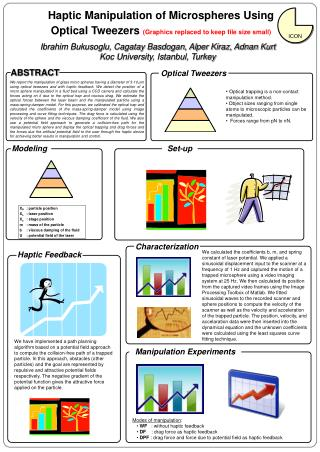DownloadDownload PresentationOptical Tweezers

# Optical Tweezers

Download Presentation## Optical Tweezers

- - - - - - - - - - - - - - - - - - - - - - - - - - - E N D - - - - - - - - - - - - - - - - - - - - - - - - - - -
##### Presentation Transcript

1. ABSTRACT Optical Tweezers Haptic Manipulation of MicrospheresUsing Optical Tweezers(Graphics replaced to keep file size small) ICON Ibrahim Bukusoglu, Cagatay Basdogan, Alper Kiraz, Adnan Kurt Koc University, Istanbul, Turkey We report the manipulation of glass microspheres having a diameter of 3-10 μm using optical tweezers and with haptic feedback. We detect the position of a microsphere manipulated in a fluid bed using a CCD camera and calculate the forces acting on it due to the optical trap and viscous drag. We estimate the optical forces between the laser beam and the manipulated particle using a mass-spring-damper model. For this purpose, we calibrated the optical trap and calculated the coefficients of the mass-spring-damper model using image processing and curve fitting techniques. The drag force is calculated using the velocity of the sphere and the viscous damping coefficient of the fluid. We also use a potential field approach to generate a collision-free path for the manipulated microsphere and display the optical trapping and drag forces and the forces due the artificial potential field to the user through the haptic device for achieving better results in manipulation and control. • Optical trapping is a non-contact manipulation method. • Object sizes ranging from single atoms to microscopic particles can be manipulated. • Forces range from pN to nN. Modeling Set-up Set-up Characterization We calculated the coefficients b, m, and spring constant of laser potential. We applied a sinusoidal displacement input to the scanner at a frequency of 1 Hz and captured the motion of a trapped microsphere using a video imaging system at 25 Hz. We then calculated its position from the captured video frames using the Image Processing Toolbox of Matlab. We fitted sinusoidal waves to the recorded scanner and sphere positions to compute the velocity of the scanner as well as the velocity and acceleration of the trapped particle. The position, velocity, and accelaration data were then inserted into the dynamical equation and the unknown coefficients were calculated using the least squares curve fitting technique. Haptic Feedback We have implemented a path planning algorithm based on a potential field approach to compute the collision-free path of a trapped particle. In this approach, obstacles (other particles) and the goal are represented by repulsive and attractive potential fields respectively. The negative gradient of the potential function gives the attractive force applied on the particle. Manipulation Experiments • Modes of manipulation: • WF : without haptic feedback • DF : drag force as haptic feedback • DPF : drag force and force due to potential field as haptic feedback